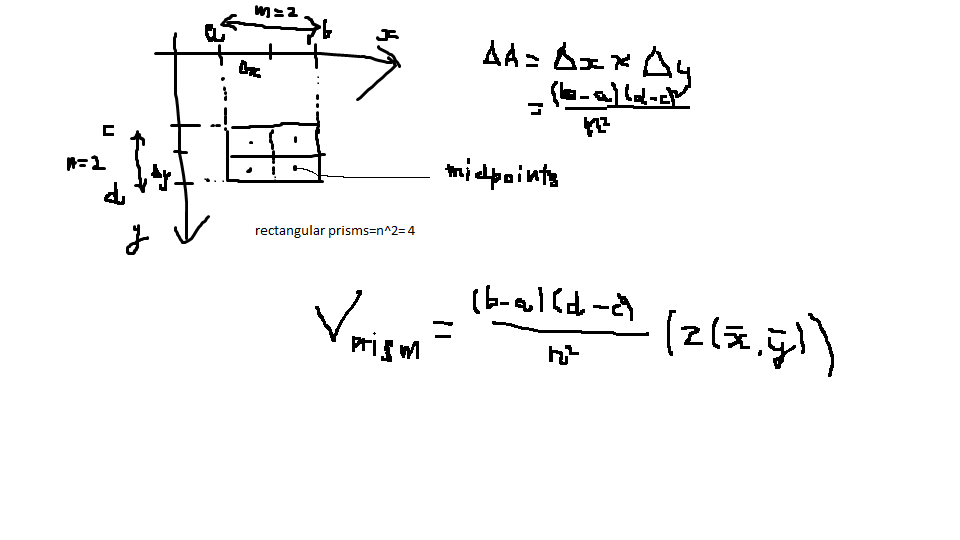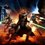# Numerical integration: double integrals with the Midpoint Rule

The Midpoint Rule is an elementary Numerical Integration iterative process. Double integrals can be calculated analytically, and numerically. The idea of the Midpoint Rule is to try and break up the integral into individual rectangular prisms and add up the individual volumes of the prisms, obtaining the approximate final volume of the double integral. Much like its two dimensional single variable counterpart, you are finding the volume under the surface, like finding the area under a curve, and instead of area approximation through rectangles, you are approximating the volume through rectangular prisms.Here is my code for the definite integral of z=x^2+y^3, choose your bounds of integration:

https://repl.it/@PhysicsAndMath/numerical-integration-double-integralsNote by Kao Cen Darach
7 months, 3 weeks ago

This discussion board is a place to discuss our Daily Challenges and the math and science related to those challenges. Explanations are more than just a solution — they should explain the steps and thinking strategies that you used to obtain the solution. Comments should further the discussion of math and science.

When posting on Brilliant:

• Use the emojis to react to an explanation, whether you're congratulating a job well done , or just really confused .
• Ask specific questions about the challenge or the steps in somebody's explanation. Well-posed questions can add a lot to the discussion, but posting "I don't understand!" doesn't help anyone.
• Try to contribute something new to the discussion, whether it is an extension, generalization or other idea related to the challenge.

MarkdownAppears as
*italics* or _italics_ italics
**bold** or __bold__ bold
- bulleted- list
• bulleted
• list
1. numbered2. list
1. numbered
2. list
Note: you must add a full line of space before and after lists for them to show up correctly
paragraph 1paragraph 2

paragraph 1

paragraph 2

[example link](https://brilliant.org)example link
> This is a quote
This is a quote
    # I indented these lines
# 4 spaces, and now they show
# up as a code block.

print "hello world"
# I indented these lines
# 4 spaces, and now they show
# up as a code block.

print "hello world"
MathAppears as
Remember to wrap math in $$ ... $$ or $ ... $ to ensure proper formatting.
2 \times 3 $2 \times 3$
2^{34} $2^{34}$
a_{i-1} $a_{i-1}$
\frac{2}{3} $\frac{2}{3}$
\sqrt{2} $\sqrt{2}$
\sum_{i=1}^3 $\sum_{i=1}^3$
\sin \theta $\sin \theta$
\boxed{123} $\boxed{123}$

Sort by:

You could post this in the calculus section as a problem

- 3 weeks, 4 days ago

Sure! Did the code work?

- 3 weeks, 2 days ago

I didn't try your code, but I will write my own if you post a problem on this

- 3 weeks, 2 days ago

I have now created a problem; please report it or let me know if anything's wrong. Thank you!

- 3 weeks ago

Hi, thanks for the recommendation! If you are a student like me and need help with homework, then go to this educational platform and find more helpful hints and save time several times on your homework.

- 1 month, 1 week ago

Hey buddy, I gave you NO RECOMMENDATION which you so talk about. Get off Brilliant and stop posting ads

- 4 weeks, 1 day ago